# Build Checkpoint 3

## Learning Gaps and Goals

At present, I am comfortable explaining what the definition of machine learning is and the difference between supervised and unsupervised learning. I also understand the K-nearest algorithm. However, I do not know much about the mechanics (or how the maths side of machine learning works.) As part of the learning, my goal is to fill this gap by learning about how machine learning works in terms of maths. I would also like to be able to use the Colab notebook and tweak the machine learning parameters. This would mean that for the right use cases/business cases, I can provide a machine learning service through Colab notebook.

## Supporting Evidence – Curation of Successes and Failures

For the above homework, I have received feedback that although my explanation on the confusion matrix is very comprehensive, the explanation of the machine learning validation pipeline seemed incomplete. This was a failure on my part. I had to comment on the code in the Colab extensively with what I learned. In fact, the machine learning pipeline part was the most useful technical knowledge I gained through the exercise. Hence, I will unpack here what I learned.

The above part of the pipeline is defining and compiling the deep neural net model.

You can see there are two HIDDEN_UNITS_LAYER. Units(represented by a number) here are representing how many neurons are in the layer. Having only two hidden layers for this model is fine to train based on a very small set of csv file. However, if the problem was image recognition, we may need more hidden layers and possibly more neurons. LEARNING_RATE is a hyperparameter that controls how much to change the model in response to the estimated error each time the model weights are updated. (Brownlee, 2019). Hyperparameter is a parameter that controls the learning process. Hyperparameter is distinct from a parameter in that it cannot be derived from training. If learning_rate is too large, it makes the model unstable as you can imagine. REGULARIZATION_STRENGTH represents applying a penalty to increasing the magnitude of parameter values in order to reduce overfitting. Overfitting occurs when the model perfectly learns a training dataset including its irrelevant noise. Learning a bit of noise is good because it prepares the model to be flexible. However, an overfitted model is not so useful as it will not recognise useful and correct patterns in newer datasets. Regularization is the technique that discourages learning a more complex or flexible mode, so that the model does not become overfit. You could think regularization is a form of regression, recognising noise in the training dataset, and shrinking or regularizing the learned coefficient estimates towards zero. model = tf.keras.Sequential tells me that the code is using Sequential API method. Broadly there are two methods available, sequential and functional.

https://towardsdatascience.com/regularization-in-machine-learning-76441ddcf99a

## Documentation

Neuron is specifically a thing that holds a number between 0 and 1. It is really nothing more than that.

Machine learning in essence is an applied calculus. It basically comes down finding a minimum of a certain function.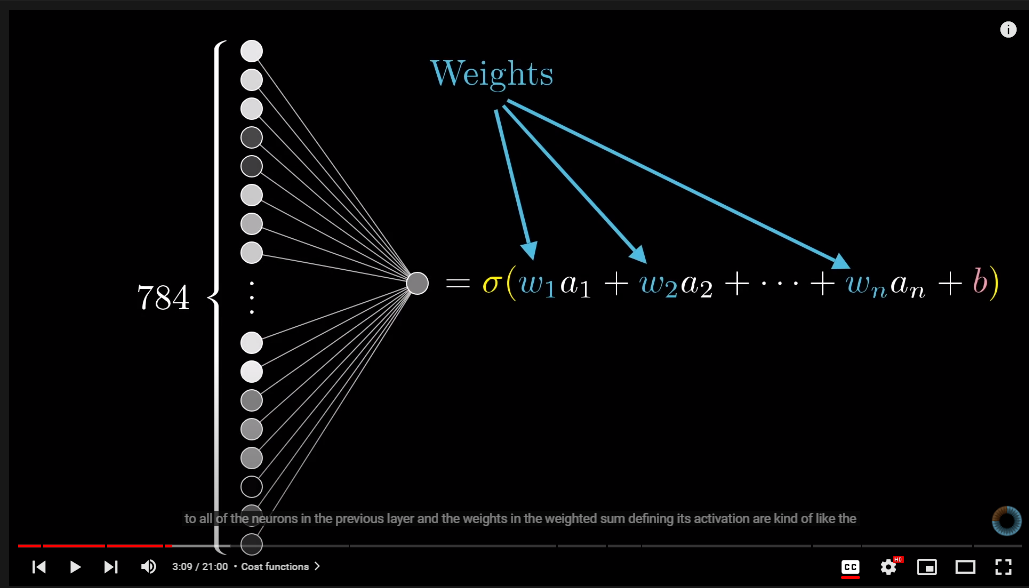Conceptually each neuron is connected to all the neurons in the previous layer.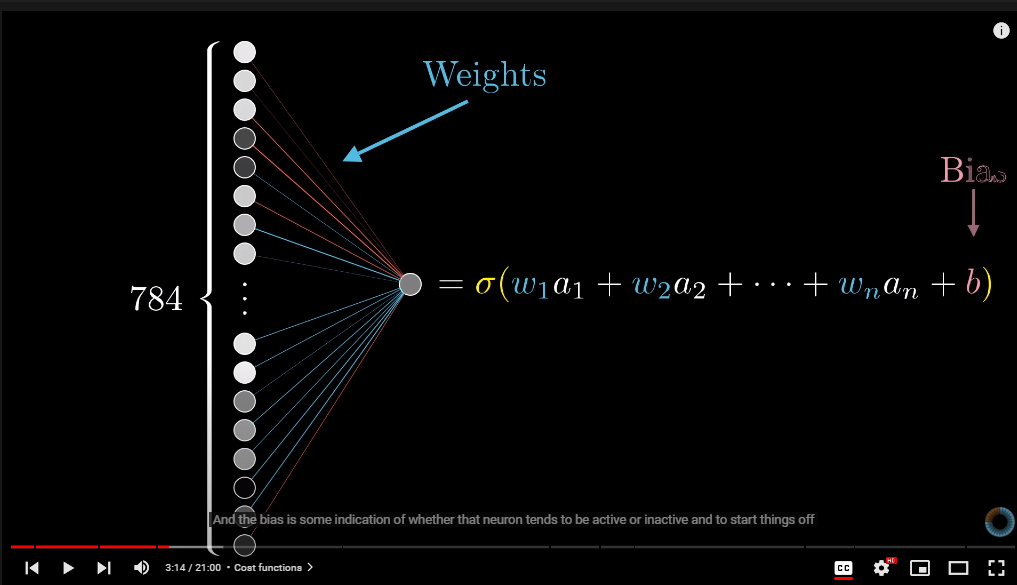Weights are kind of like the strengths of those connections and bias is a sort of indication of whether that neuron is active or inactive.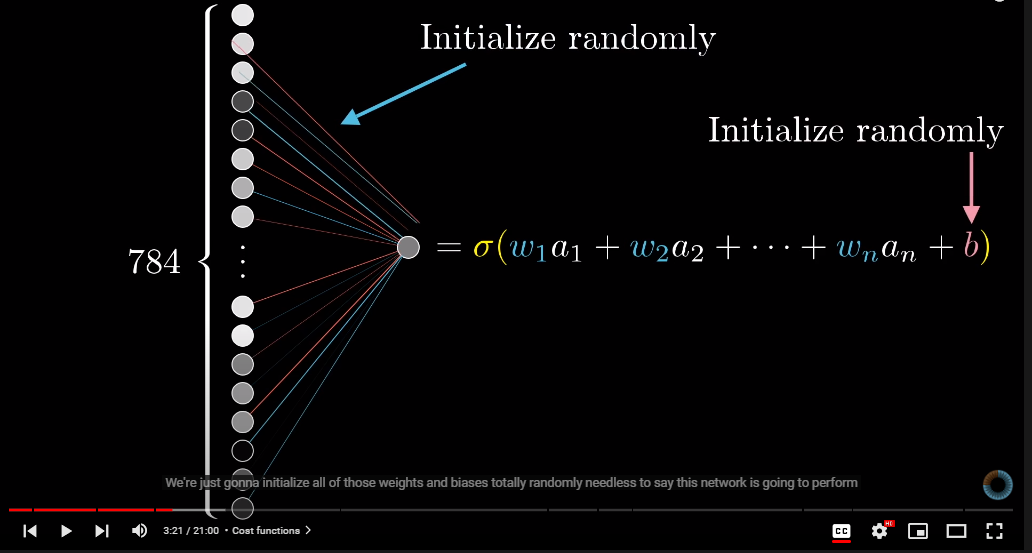And to start things off, we are just gonna initialise those variables (weights and biases) totally randomly. This randomisation means that the neural network is going to perform poorly to begin with.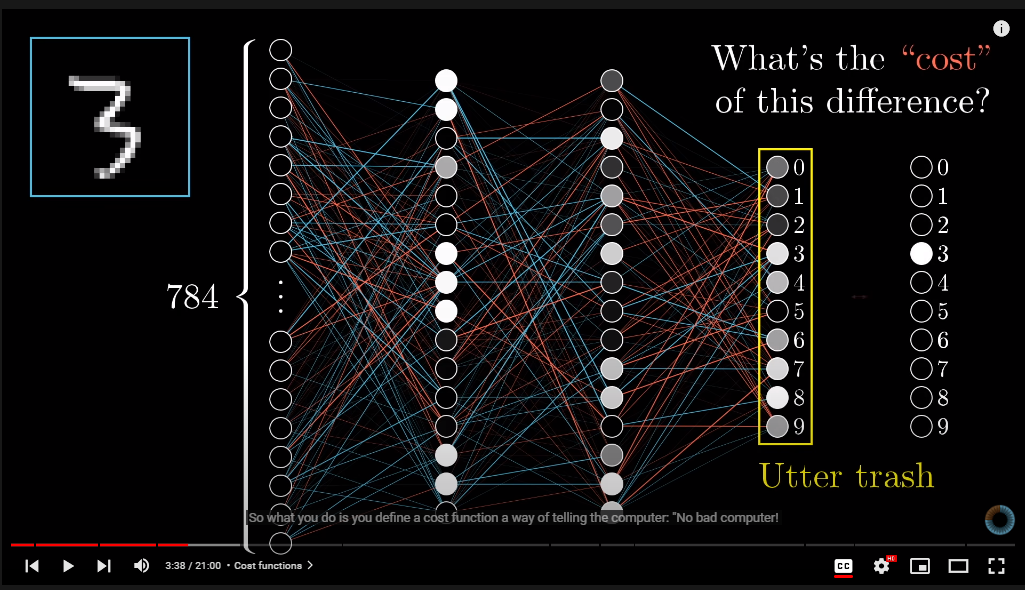So then, we define a cost function.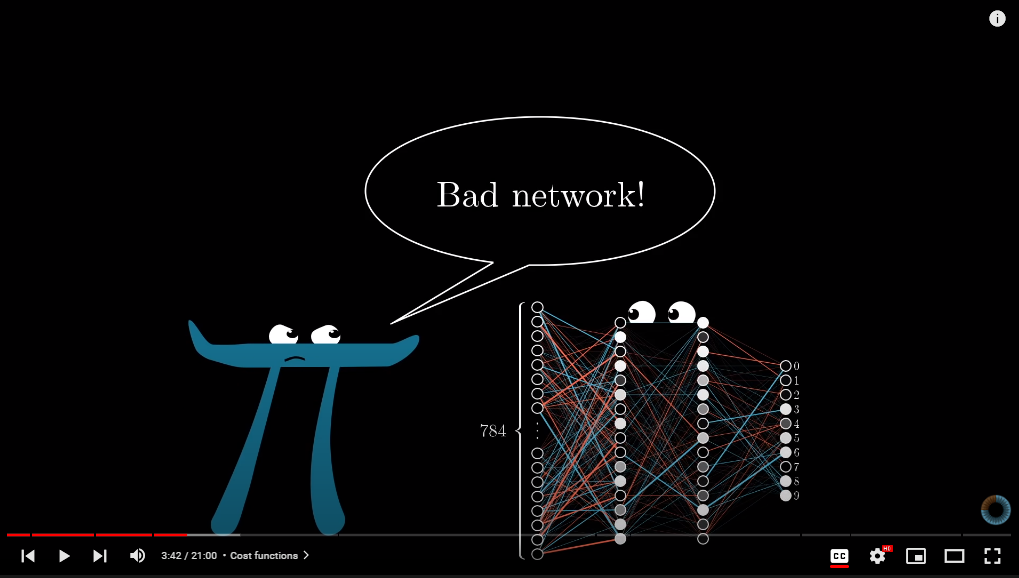It is giving the machine some sort of feedback.Add up the squares of the differences of the trash outputs and the values that I want them to have. This is what we call the cost of single training example. THis sum is small, when the network confidently classifies the images correctly, but it will be large, when the network does not know what it is doing.Then you calculate the average cost of all the tens of thousands of data.

With neural network, we don’t instruct the computer what to do. We do not break the problem down with logic for the computer. Instead, the computer learns by observing data, figuring out its own solution.

A good example of problems that regular coding find difficult to solve is image recognition.We as humans understand that all three figures look naturally like the number 3.

To code a program with logic how to recognice these images as 3 is a complicated problem.

Neural network makes this possible. It lets the computer ‘learn’ from a large number of mages labelled 3, and eventually recognice an image that is most likely to be 3 as 3. So the computer gets trained to infer rules or logic to recognise a similar image. This means the more training images (data) you provide to the computer, the better the computer’s way of recognising (model) becomes.

Understanding perceptrons is a good place to start to understand machine learning. Perceptron is a device that makes decisions by giving weights to the various factors. Suppose we are making a decision about whether or not to go to Sydney for the weekend. The following factors may be considered.

1. Is this a long weekend?
2. Is my friend that I want to catch up in Sydney free that weekend?
3. Do I not have a fun party that I am invited this weekend in Canberra?
4. Do I have a free accommodation that week in Sydney?
5. Do I not have an assignment due this weekend?

So you can think of perceptron as a decision tree that is weighted differently in each branch. The larger the weight, the more important the factor is to you.

I can choose a W1=3, W2=1, W3=3, W4=6, W5=7. This means whether or not I have an assignment due this weekend is the most important factor, and whether or not I have a friend who may hang out with me in Sydney is the least important factor. Let’s say I choose athreashold of 5 for the perceptron. This means, now, the weight equal or smaller than 5 does not affect the decision made by the perceptron. Hence, only the factor 4 and 5 are considered by the perceptron. So if I have a free accommodation in Sydney that weekend, the output of the perceptron would be 1, as an example.

I can change the weights and the threshold in the above model. This would give me different sets of outputs. If I lower the threshold to 2, then all factors would be considered except the factor number 3.

In the first layer of perceptrons, the most simple decisions are made, then in the second layer, the model weighs the results from the first layer. The second layer is making more complex and more abstract level of decisions than the first layer. Notice there is only one output.

The above description can be mathematically simplified as follows:

W refers to the vector containing weights, and x refers to the vector containing inputs.

The threshold we described above can be notated as -bias. In other words, bias is equal to -threshold. Bias is a measure of how easy it is to get the perceptron to output a 1.

http://neuralnetworksanddeeplearning.com/chap1.html

Using bias rather threshold makes the whole mathematical formula simpler in machine learning. This is a brilliant lesson for me personally. Whenever I hear the word bias in neural networks, I naturally think of the English meaning of bias. But it is important to realise that bias in the machine learning notation is just a negative threshold which means how likely is the machine output 1. (between values 0 and 1)

So again, in short, whenever machine learning ‘bias’ is mentioned, it simply means a negative threshold.

## Application of Cybernetic techniques, tools, and resources

Cybernetic thinking permeates diverse fields such as biology, neurology, sociology, ecology, economics, politics, psychoanalysis, linguistics, and computer science. (Pias, 2016) u

Machine learning in the last couple of decades has been embedded into our lives by the means of automatic insurance quotes, mortgage applications, prediction of recidivism of criminals, etc. This means most people in developed societies are affected by the decisions made by machine learning algorithms. And yet, very few people in society understand the algorithm. Further, there is no way for the people whose lives are influenced by machine learning to give feedback back to the machine learning algorithm. In this regard, the least a cybernetician could do is have a fundamental understanding of how machine learning works. This is not to say, learn to use the ml libraries when coding, but more about a solid understanding of the core principles of neural networks and deep learning. This is imperative, because if one understands the core ideas well, then he can rapidly grasp new materials and apply them. For these reasons, as a budding cybernetician, I had benefited greatly from learning the core principles of neural networks.

## References

Pias, Claus (ed.), 2016, Cybernetics: the Macy Conferences 1946-1953 : transactions, Diaphanes, Zurich.

*All the links to the websites and videos are self-explanatory above.